#MathCS.org - Statistics

## 4.4 Measures of Variability: Range, Variance, and Standard Deviation

Example: Suppose two machines produce nails which are on average 10 inches long. A sample of 11 nails is selected from each machine.

• Machine A: 6, 8, 8, 10, 10, 10, 10, 10, 12, 12, 14
• Machine B: 6, 6, 6, 8, 8, 10, 12, 12, 14, 14, 14

To verify, let's compute the mean:

• mean for machine A: 110 / 11 = 10
• mean for machine B: 110 / 11 = 10

In both cases, the mean is 10, indeed. However, the first machine seems to be the better one, since most nails are close to 10 inches. Therefore:

#### The Range

The easiest measure of the data spread is the range. It is simply the highest data value minus the lowest data value (we have seen the range before). In the above example, the range is the same for both data, namely 14 - 6 = 8. The range is, while useful, too crude a measure of variability.

#### The Variance

We want to find out how much the data points are spread around the mean. To do that, we could find the difference between each data point and the mean, and average these differences. However, we want to measure the differences to the mean regardless of the sign (positive or negative difference). Therefore, we could find the absolute value of the difference between each data point and average that. But for theoretical reasons an absolute value function is not easy to deal with, so that one chooses a square function instead (which also neutralizes signs). Finally, for yet other theoretical reasons we shall use not the sample size n to compute an average, but instead n-1.

Hence, we will use this formula to compute the data spread, or variance:

Variance = add up the squares of (Data points - mean), then divide that sum by (n - 1)

There are two symbols for the variance, just as for the mean:

•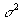is the variance for a population
•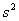is the variance for a sample

In other words, the variance is computed according to the formulas:

•(for the population variance)
•(for the sample variance)

We had to use two formulas because one involves the population mean, the other the sample mean. Practically, however, the formula is the same. It is useful to compute the variance at least once "by hand" before we show how to use Excel to accomplish the same feat quickly and easily.

#### How to find the variance "by hand":

1. Make a table of all x values
2. Find the mean of the data
3. Include a column with the difference to the mean
4. Include a column with the square of difference to the mean
5. Add the last column and divide the sum by (n - 1).

Here is the table that this procedure produces for the above sample of nails from machine A and B:

Machine A:

 x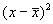6 4 16 8 2 4 8 2 4 10 0 0 10 0 0 10 0 0 10 0 0 10 0 0 12 -2 4 12 -2 4 14 4 16

Therefore, the variance for machine A is: (16 + 4 + 4 + 0 + 0 + 0 + 0 + 0 + 4 + 4 + 16) / 10 = 48 / 10 = 4.8

Machine B:

 x6 4 16 6 4 16 6 4 16 8 2 4 8 2 4 10 0 0 12 -2 4 12 -2 4 14 -4 16 14 -4 16 14 -4 16

Therefore, the variance for machine B is: (16 + 16 + 16 + 4 + 4 + 0 + 4 + 4 + 16 + 16 + 16) / 10 = 112 / 10 = 11.2

In other words, the variance, or spread around the mean, for machine A is 4.8 while machine B has a variance (spread) of 11.2. That means that machine A, as a rule, produces nails that stick pretty close to the average nail length. Machine B, on the other hand, produces nails with more variability that machine A. Therefore, Machine A would be much preferred over machine B.

Note: The unit of the variance is the square of the original unit; hence, it is not the best number (considering units). Therefore, one introduces an additional number, called the standard deviation:

#### The Standard Deviation

The standard deviation is the square root of the variance.

As with the mean, there are two letters for variance and standard deviation:

•is the variance for a population andis the population standard deviation
•is the variance for a sample andis the sample standard deviation

Example: Consider the sample data 6, 7, 5, 3, 4. Compute the standard deviation for that data.

To compute the standard deviation, we must first compute the mean, then the variance, and finally we can take the square root to obtain the standard deviation. In this case we do not need to create a table since there are so few numbers:

• Computing the mean: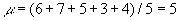• Computing the variance: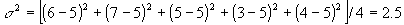• Standard deviation: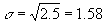#### Short-Cut for Variance

There is a nice short-cut to compute the variance that can be proved as an exercise: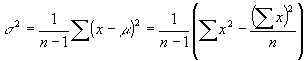At first this second formula looks much more complicated, but it is actually easier since it does not involve computing the mean first. In other words, using the second formula we can compute the variance (and therefore the standard deviation) without first having to compute the mean.

In our above example of machine B we would compute the variance using this shortcut as follows:

 x x2 6 36 6 36 6 36 8 64 8 64 10 100 12 144 12 144 14 196 14 196 14 196 sum(x) = 110 sum(x2) = 1212

Therefore the variance is:

1/ (11 - 1) * (1212 - 1102/11) = 0.1 * (1212 - 1100) = 11.2

which of course is the same number as before, but a little easier to arrive at. However, Excel - as usual - provides built-in function to compute the range, the variance, and the standard deviation.

If you need to compute the variance manually, you should always use this shortcut formula.

#### Using Excel to compute Range, Variance, and Standard Deviation

Excel provides simple formulas to compute the range, the variance, and the standard deviation:

• to compute the range: "=max(RANGE) - min(RANGE)"
• to compute the variance: "=var(RANGE)"
• to compute the standard deviation: "=stdev(RANGE)"

Example: Use the above formulas to compute the mean, the range, the variance, and the standard deviation of the salaries of graduates for the University of Florida. The data set (in Excel format) can be obtained by using the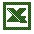University of Florida Salary Levels data set we utilized beore.

All that is involved here is adding the appropriate formulas to the Excel worksheet. The results (including the formulas) are displayed below: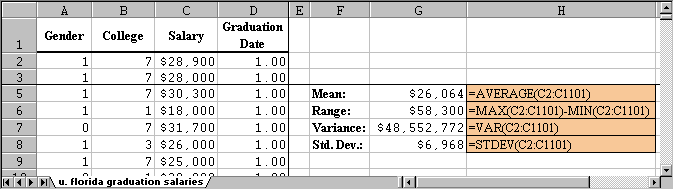Note: The variance is displayed as dollars, even though that is not correct. The correct unit for the variance, of course, is "square dollars" which does not make much sense. The standard deviation, on the other hand, has indeed dollars as unit.

#### Variance and Standard Deviation for Frequency Tables

Just as we were able to approximate the mean and median of a variable from its distribution (frequency table or histogram) we can do something similar for the variance (and hence the standard deviation).

Example: A study of salaries of graduates from a University shows their income as follows:

 Salary Range Count \$7,200 - \$18,860 130 \$18,860 - \$30,520 698 \$30,520 - \$42,180 254 \$42,180 - \$53,840 16 \$53,840 - \$65,500 2

Estimate the standard deviation for this variable. Hint: you may use the following table (of course together with Excel) to get organized. If you have trouble understanding this table, check the video below, explaining a slightly easier example.

 Salary Range count mid count*mid mid2 cont*mid2 \$7,200 - \$18,860 130 13,030 1,693,900 169,780,900 22,071,517,000 \$18,860 - \$30,520 698 24,690 17,233,620 609,596,100 425,498,077,800 \$30,520 - \$42,180 254 36,350 9,232,900 1,321,322,500 335,615,915,000 \$42,180 - \$53,840 16 48,010 768,160 2,304,960,100 3,687,841,600 \$53,840 - \$65,500 2 59,670 119,340 3,560,508,900 7,121,017,800 Total 1,100 29,047,920 793,994,369,200

To estimate the variance we use the shortcut formula:

1/(n-1)*(sum of squares - (sum)2/N) =
= 1/1099*(793994369200 - 290479202/1100 =
= 1/1099 * 60111656176 = 54696684.42

Thus, the variance is approximately 54,696,684.42 and thus the standard deviation is the square root of that number, or \$7,395.72. That is close enough to the true values computed previously.

The numbers in this example turned out to be huge, which made the process somewhat confusing. For smaller numbers, everything seems a little bit easier, hopefully.

Example:  The evaluation of a statistics lecture resulted in the following frequency distribution. Find the mean, median, variance, and standard deviation.

 Category Count very good 10 good 5 neutral 4 poor 2 very poor 1